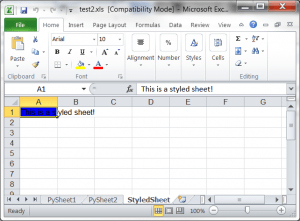# Creating Microsoft Excel Spreadsheets with Python and xlwt

There are a couple of ways to create Microsoft Excel spreadsheets with Python. You can use PyWin32’s win32com.client method, which was discussed in an old article a number of years ago or you could use the xlwt package. We’ll be looking at the latter in this article. You will learn how to create an Excel spreadsheet with multiple worksheets and how to create styled cells. Let’s get started!

### Getting xlwt

You will want to go and download xlwt so you can follow along. It is available on PyPI. If you have pip installed, then you can install it that way as well. Once you have the module, we will be ready to continue.

### Using xlwtThe xlwt package is pretty easy to use, although the documentation is a bit sparse. On the plus side, the xlwt Github repository has lots of examples. Let’s create a simple Excel spreadsheet with a single worksheet:

```import xlwt

#----------------------------------------------------------------------
def main():
""""""
book = xlwt.Workbook()

cols = ["A", "B", "C", "D", "E"]
txt = "Row %s, Col %s"

for num in range(5):
row = sheet1.row(num)
for index, col in enumerate(cols):
value = txt % (num+1, col)
row.write(index, value)

book.save("test.xls")

#----------------------------------------------------------------------
if __name__ == "__main__":
main()
```

Let’s break this down a bit. First off, we import the xlwt module and create a function called main. In the main function, we create an instance of xlwt.Workbook and add a worksheet via the add_sheet method. We label out worksheet with the text “PySheet1”. Then we create a nested loop to create 5 rows and 5 columns worth of content. Basically we write the Row / Column information to each cell. Then we save the file and we’re done!

### Creating a Styled CellNow let’s refactor the code in such a way that we can add worksheets by using a function. We’ll also create another function that can create a worksheet with a styled cell:

```import xlwt

#----------------------------------------------------------------------
"""
Add a sheet with one line of data
"""
value = "This sheet is named: %s" % name
sheet.write(0,0, value)

#----------------------------------------------------------------------
"""
"""
value = "This is a styled sheet!"
style = 'pattern: pattern solid, fore_colour blue;'
sheet.row(0).write(0, value, xlwt.Style.easyxf(style))

#----------------------------------------------------------------------
def main():
""""""
book = xlwt.Workbook()

cols = ["A", "B", "C", "D", "E"]
txt = "Row %s, Col %s"

for num in range(5):
row = sheet1.row(num)
for index, col in enumerate(cols):
value = txt % (num+1, col)
row.write(index, value)

book.save("test2.xls")

#----------------------------------------------------------------------
if __name__ == "__main__":
main()
```

Here we create an add_sheet method that accepts a Workbook instance and the name of the sheet. It will add a worksheet to the book with a cell that identifies what the name of the worksheet is. The add_styled_sheet works in much the same way except that it creates a styled cell with a message.

### Creating a More Complex Styled CellIn this example, we will use the original code to create the same 5×5 set of cells. Then we’ll also create a cell with a red background, a border and specific date format:

```from datetime import date
import xlwt

#----------------------------------------------------------------------
def main():
""""""
book = xlwt.Workbook()

cols = ["A", "B", "C", "D", "E"]
txt = "Row %s, Col %s"

for num in range(5):
row = sheet1.row(num)
for index, col in enumerate(cols):
value = txt % (num+1, col)
row.write(index, value)

value = date(2009,3,18)
fmt = xlwt.Style.easyxf("""
font: name Arial;
borders: left thick, right thick, top thick, bottom thick;
pattern: pattern solid, fore_colour red;
""", num_format_str='YYYY-MM-DD')
sheet1.write(6,1,value, fmt)

book.save("test3.xls")

#----------------------------------------------------------------------
if __name__ == "__main__":
main()
```

Here we use a large string to specify to xlwt that we want to apply a style that uses the Arial font, has borders on all four sides of the cell and the fore_color is red. When you execute the code, you will find that fore_color actually means background color. Anyway, this syntax makes it very easy to style a cell’s contents. There are a lot of good examples in this PDF that the Python Excel website put out.

### Wrapping Up

Now you know how to use the xlwt package to create simple Microsoft Excel spreadsheets. You will notice that it create the spreadsheets using the older *.xls format. The xlwt module does not currently support the *.xlsx format. For that, you would probably have to work with PyWin32 or possibly the openpyxl project or XlsxWriter. Good luck and happy coding!